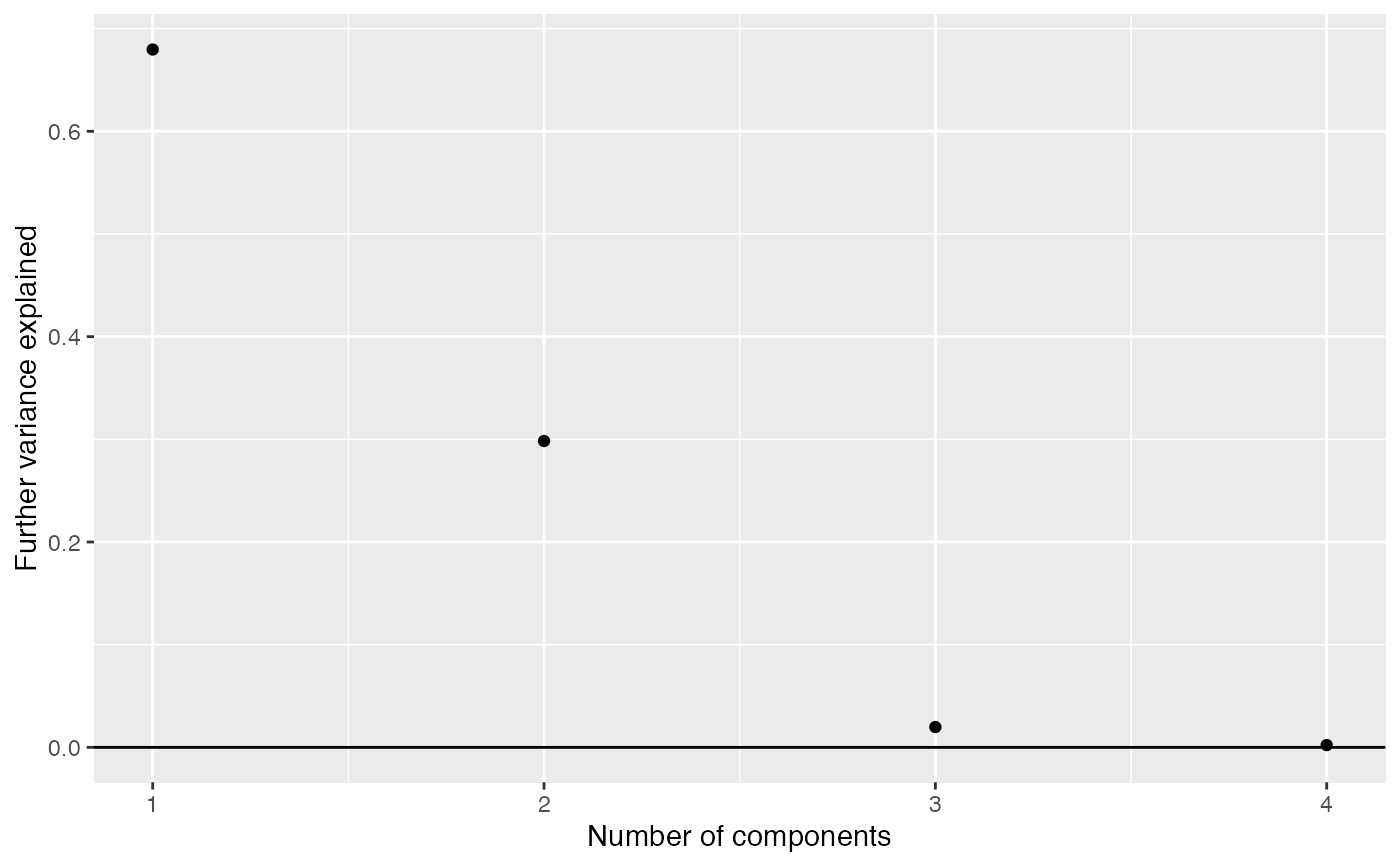Based on the output of components_seq, work out how much further variance is explained by adding further components.

components_seq_scree(comp_seq, rand_comp = NULL)

components_seq_screeplot(comp_seq, rand_comp = NULL)

## Arguments

comp_seq A list of Components objects, as produced by components_seq. Optional. A Components object with a single component. This should be based on a randomized version of the weitrix, for example as produced by weitrix_components(weitrix_randomize(my_weitrix), p=1).

## Value

components_seq_scree returns a data frame listing the variance explained by each further component.

components_seq_screeplot returns a ggplot2 plot object.

## Details

If rand_comp is given, some possible threshold levels for including further components are also calculated.

The "Parallel analysis" threshold is chosen based on varianced explained by a single component in a randomized weitrix.

The "Optimistic" thresholds are chosen starting from the "Parallel Analysis" threshold. We view the Parallel Analysis threshold as indicating random variance is split amongst an effective number of samples, which will be somewhat smaller than the real number of samples. As each component is accepted, the pool of remaining variance is reduced by its contribution, and also the number of effective samples is reduced by one. The threshold is then the size of the remaining variance pool divided by the effective remaining number of samples. This is a somewhat ad-hoc method, but may indicate more components are justified than by criteria based on a flat threshold.

## Examples

comp_seq <- weitrix_components_seq(simwei, 4, verbose=FALSE)

components_seq_scree(comp_seq)
#>   components   explained
#> 1          1 0.679650550
#> 2          2 0.298364357
#> 3          3 0.019773284
#> 4          4 0.002211809components_seq_screeplot(comp_seq)